```{ subscribe_url:'/share/sites/library-of-congress-blogs/geography-and-maps.php' }```

# Computing Space I: Ernesto and Kathy Split a Sandwich

This post is dedicated to the memory of  Katherine Kiernan, one of the only female programmers at the Harvard Laboratory for Computer Graphics and Spatial Analysis, during the early years of the development of Geographical Information Systems. She passed away last year.

Today’s post is the beginning of a series called,”Computing Space,” which will highlight the lives and work of many of the mostly unknown cartographers, geographers, mathematicians, computer scientists, designers and architects who had a hand in the birth of today’s computer cartography.

–If the intended application of mathematics is essential, how about parts of mathematics whose application—or at least what mathematicians take for their application—is quite fantastic?   —Ludwig Wittgenstein

Given any three sets in space, each of finite outer Lebesque measure, there exists a plane which bisects all three sets, in the sense that the part of each set which lies on one side of the plane has the same outer measure as the part of the same set which lies on the other side of the plane.

The above statement of the Ham Sandwich Theorem, which is a classic mathematical proof in the field of algebraic geometry, is written at the beginning of William Warntz’ introduction to The Sandwich Theorem: A basic one for geography, published by the Harvard Laboratory for Computer Graphics and Spatial Analysis, in 1971. To most people, the complexity of the theorem’s language hardly seems, at least at first reading, to have any importance to the history of cartography or to mapmaking as it is generally known.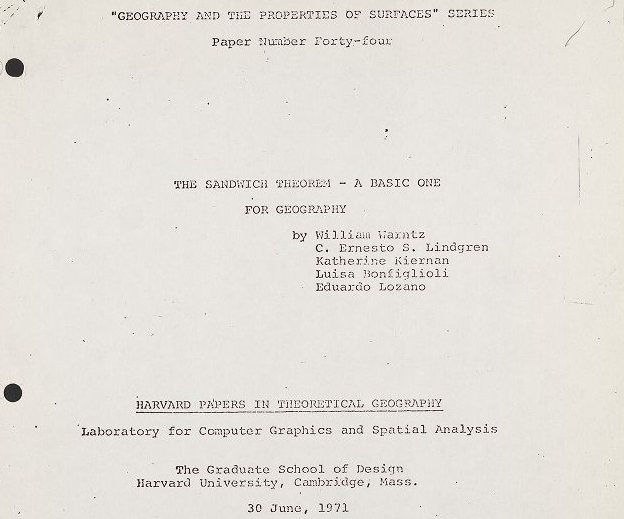Title Page of the Sandwich Theorem: a basic one for geography. Geography and Map Division, Library of Congress

In the beginnings of computer cartography however, there where many mathematicians, computer scientists, and a small group of extremely creative designers and architects who thought otherwise. William Warntz, who was the director of the Harvard Lab, described the theorem as of “paramount importance” to the field of geography and mapmaking. Researchers, like Ernesto Lindgren, Katherine Kiernan, Luisa Bonfigliolo and Stephen Selkowitz, names that are hardly household, and today would be unknown even to most practitioners of GIS, developed imaginative geometric and graphic visualizations to many complex theorems from pure mathematics that at the time no algorithmic recipes for their solution.

In their introduction to the Sandwich Theorem paper, which was authored by Warntz, Lindgren, Kiernan and a few others, they ask us to assume the earth to be a solid sphere and to picture the infinite number of planes that one could choose to bisect its volume. After presenting this rather straightforward set of bisecting planes, the paper moves to discuss other types of partitioning of the earth’s surface that are more abstract and employ less physically imaginable variables. Warntz writes:

It is certain that there exists the possibility of finding a small circle on the earth’s surface such that two (unequal) parts into which it divides the earths surface contains half of the world’s communists, half the world’s income and half of the world’s volcanoes. Another circle (presumably a different one than above) partitions the earth’s surface into two equal shares of mosques, synagogues and cathedrals.

The idea of partitioning geographic and social variables and to mapping their distribution on the surface of the earth has always been one of the mainstays of thematic cartography and the Sandwich theorem, though highly abstract, gives hope that any group of variables that we could envision as really existing could be partitioned into “regions” of equal size.  According to the paper, spatial partitioning is extremely important to theoretical geography and hence, the Sandwich Theorem was deemed by the authors to be, “one of the most fundamental theorems for geography as it demonstrates the kinds of partitioning possible for ‘n’ sets in ‘N’ dimensions”.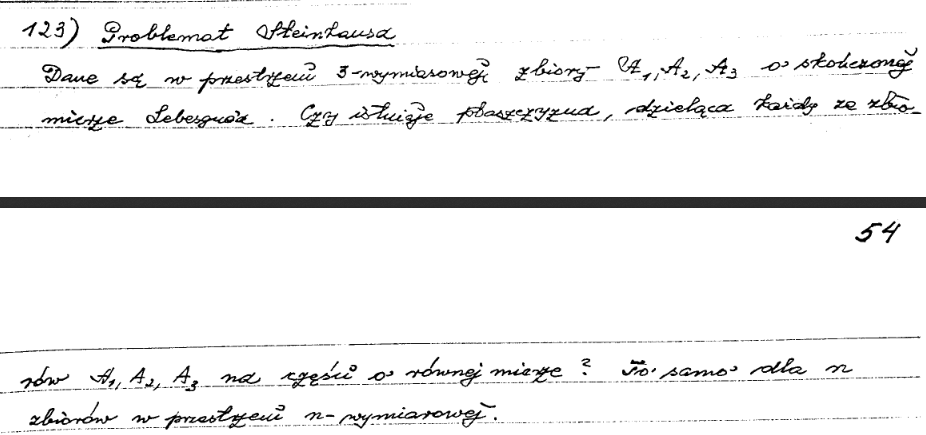Page from the manuscript of the Scottish Notebook showing problem 123. Digital image courtesy of the Archive of Wroclaw University

The origin of the partitioning problem that the Sandwich theorem tries to solve comes from what is known as the Scottish Problem Book, where it appears as problem number 123. The book was actually a large notebook used by the group of mathematicians who hung out at a place called the Scottish Cafe, in Lwow Poland, before the Second World War. Into the notebook they copied interesting problems that they proposed to each other and tried to solve. It seems the Sandwich theorem was originally laid out as a conjecture by the mathematician Stanislaus Ulam, who was part of the Lwow group, and who, during WWII emigrated to the United States to work on the Manhattan Project.

The name of the Sandwich Theorem comes from the most straightforward example of its application. If one builds a sandwich of bread, cold cuts, cheese, and then spreads it with mustard, is there a way to cut all of the elements that make up the sandwich precisely in half with a single cut of a plane? The theorem implies that the cut would produce two sections of the sandwich each of which has precisely half of the bread, half of the cold cuts and half of all of the other things at the same time no matter how they were originally oriented or distributed on the sandwich.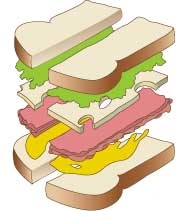The Ham Sandwich theorem was first fully explored in a paper by Hugo Steinhaus (1887-1972) and published in the journal Fundamenta Mathematicae in 1945, under the title, Sur la division des ensembles de l’espace par les plans et des esembles plans par les cercles, [On the Division of the Sets of Space by Planes of the Sets of the Planes by Circles]”. The original article is translated in full in the Sandwich Theorem paper. The theorem is part of a family of mathematical proofs called existence theorems and no matter how important Warntz thought it to be for geography and mapping, it immediately posed a problem for applications in thematic cartography.

The theorem begins with the statement, “given any three sets there EXISTS a plane”, which is a statement of existential quantification; the theorem then goes on to prove that such a plane exists in the real world. The problem with the theorem and with all existence theorems is that they provide no way to actually calculate the mathematical object that the theorem claims existence for. In pure mathematics this is not usually an issue, but for actual applications to thematic cartography, one does need to find the partition in the real world that one is claiming existence for. Existence theorems are not only problematic for cartography, but there has been a standing debate in mathematics itself as to their philosophical foundations and usefulness (for more on this see Mathieu Marion’s paper, “Kronecker’s Safe Haven for Real Mathematics“).

Warntz in his introduction calls attention to the fact that as an existence theorem the work of Steinhaus provided no analytical means for finding the partitions, but that he hoped that “numerical-graphical procedures may effect accurate approximations of the solution for given problems. Especially is computer graphic suited to such tasks.” The other parts of the Sandwich Theorem paper reflect Warntz’ hope, and take up the geometric, numerical, and cartographic solutions to the problem, culminating with a map of the United States that shows a partitioning of the three thematic variables of geographical area, population and income.

In order to get to the map, however, the abstract notions described in the theorem had to be made calculable for the available computers of the time. The question of whether this was even possible was still to be answered as no one had ever looked for an algorithmic solution to the Sandwich Theorem and general applications of existence theorems in cartography were exceedingly rare. That the members of the Harvard Lab recognized the newness of using existence theorems and the associated problems with adopting them to cartography is shown quite explicitly in a statement by Stephen Selkowitz, the author of another paper published by the Harvard Lab, which also develops an algorithmic solution to an existence theorem. In the paper, which goes by the not so catchy title, Geography and an Existence Theorem: A cartographic solution to the localization on a sphere of sets of equal-valued antipodal points for two continuous distributions with practical applications to the real earth, Selkowitz explains,

Even this relatively simple computer assistance in finding spatial solutions to the given existence theorem demonstrates the possibility of using computer mapping programs to find spatial solutions to that whole class of existence theorems having explicit or implicit spatial connotations. After initial problems related to adopting the particular existence theorem to a computer solution are resolved, computer mapping techniques are capable of quick and accurate spatial solutions with a minimum of human manipulation. This is another indication that the problem solving capabilities of computer mapping are at least as diverse and effective as are its proven capacities graphically to represent stores of spatially ordered information. These capabilities lay largely untapped, waiting to be exploited.

In part I of the Sandwich Theorem paper, “A Geometric Analysis Concerning the Sandwich Theorem,” C. Ernesto Lindgren restates the theorem in the form given by Stone and Tukey in their generalization of Steinhaus’ work, “Given any three sets in space, each of finite Lebesque measure, there exists a plane which bisects all three sets, ”and begins to speculate on its possible algorithmic simplification.

An amazing ability to intuit complex geometrical swas obviously one of Lindgren’s talents, having produced  a ground breaking book on visualizing four-dimensional Euclidean geometry. It is a book that I go back to time and time again for inspiration in my own mathematical research and in the last twenty years it has scarcely left my desk. Recently, at a conference at Harvard University, celebrating the 50th anniversary of the founding of the Harvard Laboratory in 1965, Carl Steinitz, the Alexander and Victoria Wiley Professor of Landscape Architecture, echoed my feelings when he commented that much of Lindgren’s work has yet to be developed and is critically important to the history of early spatial analysis and GIS.

After his short introduction to the theorem, Lindgren writes, “Now, for more down-to-earth applications of this possibility, we had to look for simpler approaches, not involving the required advanced mathematics, because we lack the necessary background.” Realizing the mathematical complexity of the theorem and the difficulties that it would entail to solve it analytically, he goes on to say: “Consequently, we had to begin by reasoning that this existence theorem could be stated by basing its affirmation on other conclusions, preferably not mathematical.”

In his analysis Lindgren decides to take a surface-oriented approach to the problem and starts by looking at the logical structure of the theorem and pulling it apart, “It just happens that geometry provides such possibility and, if one wished to put claim on discovery, perhaps one should recognize this priority to geometry as a whole. As it turned out, the sandwich theorem, under the light of geometric synthesis, is only a conclusion.” What Lindgren is describing here is an approach to problems that the Harvard Lab would often experiment with, namely, the development of algorithms and cartographic approaches to solving problems previously known only as results in pure mathematics by resolving the logic of the theorem into its base geometric parts.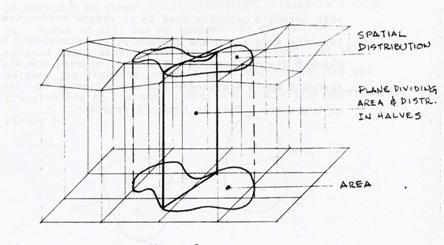Bisection of two spatial distribution by a plane. Drawing by Ernesto Lindgren for the Sandwich Theorem Paper. Geography and Map Division, Library of Congress.

Lindgren, whose main research interest at the time centered on four-dimensional geometries, used a geometric analog of angle bisection to picture the partitioning of cartographic surfaces. In figure above Lindgren describes the layering of two surface distributions and the plane that bisects them. The top layer represents schematically the surface distribution of population. The top surface in the diagram is not flat, but is curved in places to show the value of the population much like the potential surfaces found in the new population surface potential maps being developed at the time.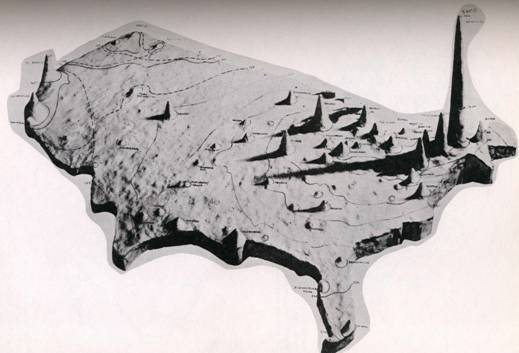Surface Population Potential Map Developed by William Warntz in 1960. Image courtesy of the US Office of Naval research, Selected Projects Conference Notes, July, 1972

The bottom distribution shown in Lindgren’s drawing represents geographic area, say, for example, of the United States or any finite region.  What follows in the paper is a complicated treatment of the notion of angle and the possible iterative solutions to the problem of bisecting all of the distributions simultaneously. Lindgren’s solution corresponds to a series of geometric constructions that divide the various distributions in half. The solution to the overall problem was found using an iterative process that calculated various partitioning schemes for each distribution until one is found that corresponds to the partition for all of them.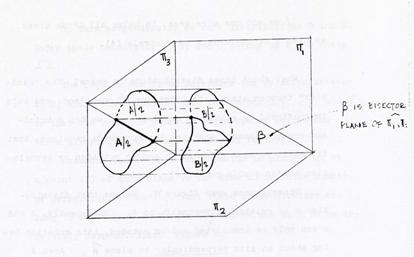Drawing by Ernesto Lindgren of the distribution as an angular bisector for the Sandwich Theorem Paper. Geography and Map Division, Library of Congress.

The iterations produced not an exact solution to the partitioning problem but an approximate one that could be made more accurate depending on the amount of computer time one was willing to spend on the calculation. The actual computer code for the program to accomplish the partitioning was authored by Katherine Kiernan, one of the only women to work at the Lab in its foundational years. In her program she used a common numerical technique of searching for a minimum by constantly reducing the space that was being analyzed. It is not known how much computer time was expended, but multiple iterations must have been necessary since there were 3,070 counties used in the calculation. The approximate nature of the solution can be seen in figure below, which is the computer printout from the Sandwich Theorem paper, and shows the percentages of the three thematic distributions that were partitioned. If this were an exact solution all the values for area, income and population would read 50%.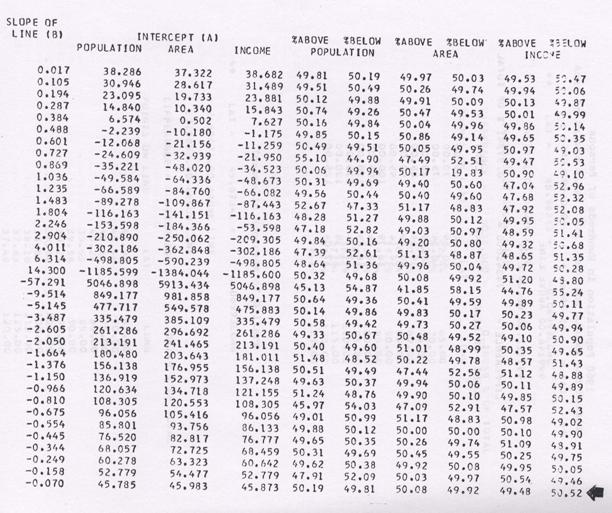Computer Printout from Katherine Kiernan’s Program Showing the Percentages of each of the partitions. Geography and Map Division, Library of Congress

The map showing graphically the calculated line dividing between the three thematic variables of population, income and area from the Sandwich Theorem paper, is shown below. Above and below the line there are simultaneously 50% of each of the each of the variables, as predicted to exist by the Sandwich theorem.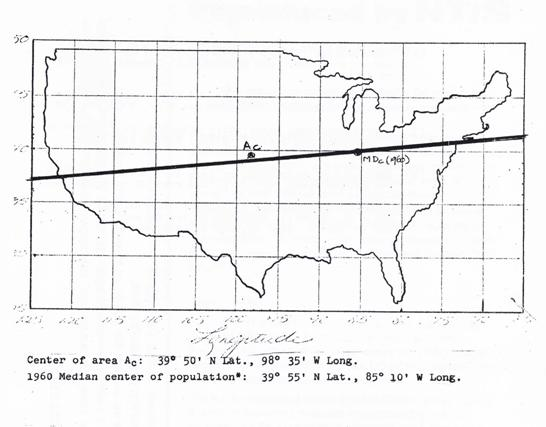Map from the Sandwich Theorem Paper showing a line that simultaneously divides area, population and income for the continental United States. Geography and Map Division, Library of Congress

Although much of what I have written about here may appear to the historian of cartography to have been a mere exercise in mathematics and programming virtuosity by two talented researchers, it had much more effect on the future of cartography and the philosophical nature of what maps would become than is generally realized. Warntz, in the preface to the Existence Theorem paper discussed in some detail the philosophical and conceptual shifts that would be brought about by this new ability to import abstract ideas from mathematics into cartographic analysis. After a description of the Borsuk-Ulam theorem, he writes about this new form cartography and mapmaking :

Theoretical geography is a science of earth location and spatial relations. It describes, classifies, and predicts locations in the spatial sense. Carto-graphics stands to geographical science as graphics does to science generally. Mapping is a geographical concept in the theory of sets.

This definition of mapping was radically innovative at the time. Cartography and mapping are no longer seen as just printed planar representations of the real world, but are algebraically defined and treated as applications of the theory of sets. Maps have become more abstract and axiomatic, but because of their ability to be algebraically manipulated, they have also become more useful tools for complex geographical analysis. This way of looking at maps was to have important implications for the development of GIS and other forms of computerized mapping. Warntz goes to predict,

Cartography is the geographical example in the application of this concept. That which is ordinarily called a map by geographers and laymen is technically “a graphical image of a mapping.” Whatever useful roles graphics plays in science generally also can be claimed for cartography with relation to geographical science. This is especially true now that most geographers increasingly employ geometry as an appropriate vehicle to carry their discipline.

Today, as we see the boundary between cartography, big data infographics, and dynamic forms of visualization is continually being blurred,  the vision of these early innovators is coming clearly into view. Warntz’ use of the word “Carto-graphics” was a glimpse into the future, one that many amazing talents had a hand in developing.

For more on the the changing view of cartography and its role in science see Pablo Ivan Azocar Fernandez, Paradigms in Cartography: an epistemological review for the 20th and 21st centuries (Berlin: Springer Verlag, 2014)